# Simultaneous Inferences and Other Regression Topics KNNL Chapter

• Slides: 12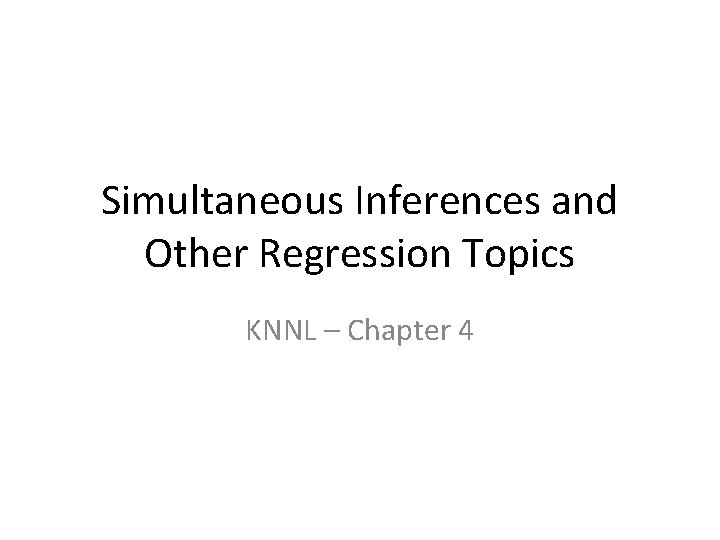Simultaneous Inferences and Other Regression Topics KNNL – Chapter 4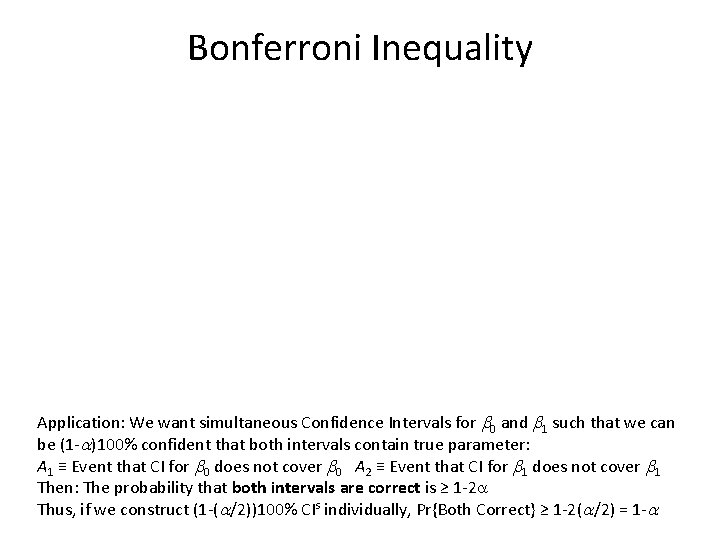Bonferroni Inequality Application: We want simultaneous Confidence Intervals for b 0 and b 1 such that we can be (1 -a)100% confident that both intervals contain true parameter: A 1 ≡ Event that CI for b 0 does not cover b 0 A 2 ≡ Event that CI for b 1 does not cover b 1 Then: The probability that both intervals are correct is ≥ 1 -2 a Thus, if we construct (1 -(a/2))100% CIs individually, Pr{Both Correct} ≥ 1 -2(a/2) = 1 -aJoint Confidence Intervals for b 0 and b 1Simultaneous Estimation of Mean Responses • Working-Hotelling Method: Confidence Band for Entire Regression Line. Can be used for any number of Confidence Intervals for means, simultaneously • Bonferroni Method: Can be used for any g Confidence Intervals for means by creating (1 -a/g)100% CIs at each of g specified X levelsBonferroni t-table (a = 0. 05, 2 -sided)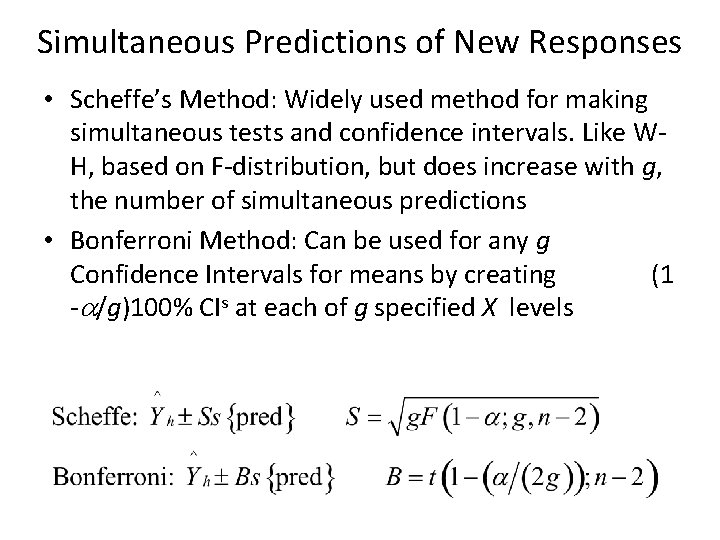Simultaneous Predictions of New Responses • Scheffe’s Method: Widely used method for making simultaneous tests and confidence intervals. Like WH, based on F-distribution, but does increase with g, the number of simultaneous predictions • Bonferroni Method: Can be used for any g Confidence Intervals for means by creating (1 -a/g)100% CIs at each of g specified X levelsRegression Through the Origin • In some applications, it is believed that the regression line goes through the origin • This implies that E{Y|X} = b 1 X (proportional relation) • Note, that if we imply that all Y=0 when X=0, then the variance of Y is 0 when X=0 (not consistent with the regression models we have fit so far) • Should only be used if there is a strong theoretical reason • Analysis of Variance and R 2 interpretation are changed. Should only use t-test for slope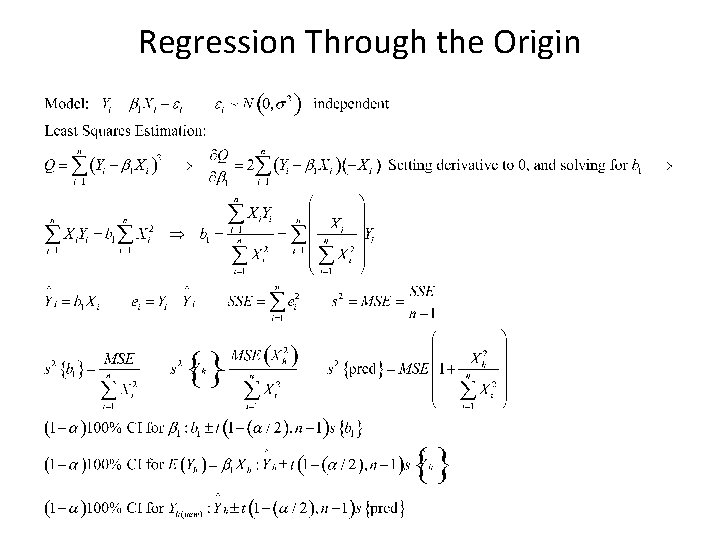Regression Through the OriginMeasurement Errors • Measurement Error in the Dependent Variable (Y): As long as there is not a bias (consistently recording too high or low), no problem (Measurement Error is absorbed into e). • Measurement Error in the Independent Variable (X): Causes problems in estimating b 1 (biases downward) when the observed (recorded) value is random. See next slide for description. • Measurement Error in the Independent Variable (X): Not a problem when the observed (recorded) value is fixed and actual value is random (e. g. temperature on oven is set at 400⁰ but actual temperature is not)Measurement Error in X (Random)Inverse Prediction/Calibration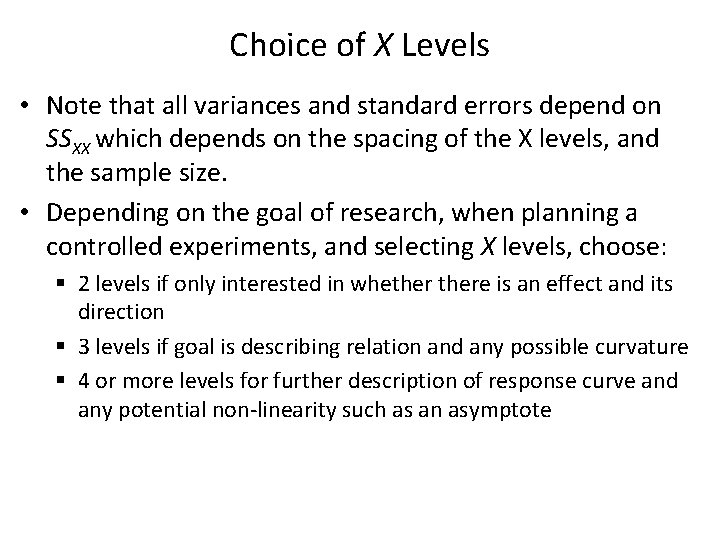Choice of X Levels • Note that all variances and standard errors depend on SSXX which depends on the spacing of the X levels, and the sample size. • Depending on the goal of research, when planning a controlled experiments, and selecting X levels, choose: § 2 levels if only interested in whethere is an effect and its direction § 3 levels if goal is describing relation and any possible curvature § 4 or more levels for further description of response curve and any potential non-linearity such as an asymptote